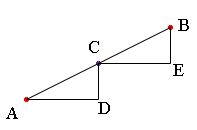SEARCH HOMEMath Central Quandaries & QueriesQuestion from JOEL, a student: A QUADRILATERAL A,B,C,D HAS THE CO -ORDINATE [2,5],[8,7],[10,3]&[0,1] RESPECTIVLY [B,E,G,H] ARE THE MID POINTS OF THE SIDES AB,BC,CD&DA RESPECTIVLY FIND THE MID POINTS OF [FG]&[FH]Joel,

Suppose you have the line segment joining A with coordinated [3, 1] and B with coordinates [5, 2], and you want to find the midpoint of this line segment. I let C be the midpoint and constructed triangles CAD and BCE using horizontal and vertical line segments.Triangles CAD and BCE are similar and |BC| = |CA| so the triangles are congruent. Thus |AD| = |CE| and hence the x-coordinate of C is midway between the x-coordinates of A and B. That is the x-coordinate of C is (5 + 3)/2 = 4. Similarly the y-coordinate of C is (2 + 1)/2 = 3/2.